# javaScript 中数组的操作

[隐藏]

## 1、length

```var arr = ["aaa","bbb"];
```

## 2、索引

```var arr = ["aaa","bbb"];
arr = "111";
```

## 3、indexOf 和 lastIndexOf

```var arr = ["aaa","bbb",111, 'bbb', 'ccc'];
```

`lastIndexOf` 的功能为返回一个指定的字符串值最后出现的位置，在一个字符串中的指定位置从后向前搜索。

```alert(arr.lastIndexOf("bbb")); // 3
```

## 4、slice

```var arr = ['A', 'B', 'C', 'D', 'E', 'F', 'G'];
alert(arr.slice(0, 3)); // 从索引0开始，到索引3结束，但不包括索引3: ['A', 'B', 'C']
alert(arr.slice(3)); // 从索引3开始到结束: ['D', 'E', 'F', 'G']
```

## 5、push

```var arr = [1,2];
```

## 6、pop

```var arr = [1,2,3];
var arr1 = [];
```

## 7、unshift

```var arr = [1,2,3];
```

## 8、shift

```var arr = [1,2,3];
```

## 9、sort

```var arr = ["3","2",11];
```

```function compare(val1,val2){
return val1-val2;
}
var arr = [3,2,9,4];
```

## 10、reverse

```var arr = ["333","222"];
```

## 11、splice

```var arr = ["a","b","c"];
```

## 12、concat

```var arr = ["a","b","c"];
```

## 13、join

```var arr = ["a","b","c"];
```

## 14、map

```var arr = [1,2,3,-1];
return x * x;
}
)); //返回1,4,9,1
```

## 15、reduce 和 reduceRight

```var arr = [1,2,3,-1];
var result = arr.reduce(function(prev,cur,index,array){
return prev+cur
})
```

reduceRight 方法的功能及参数和 reduce 是一样的，不同的是 reduceRight 从数组的末尾项开始，逐个向前遍历。

## 16、filter

```var arr = [1,-3,2,-4,-6];
var newArr = arr.filter(function(x){
if(x>=0){
return true;
}else{
return false;
}
})
```

## 17、toString

```var arr = [1,2];
```

## 18、valueOf

```var arr = [1,2];
```

## 19、toLocaleString

```var arr = [1,2];
```

## 20、some

```var arr = [2, 5, 8, 1, 4];
arr.some(item => item === 3); // false
arr.some(item => item > 3); // true
```

## 21、every

```var arr = [2, 5, 8, 1, 4];
arr.every(item => item > 3); // false
arr.every(item => item > 0); // true
```

## 22、forEach

```var arr = [1 ,3];
arr.forEach(item => console.log(item)); // 1 3
```

## 23、Array.from()

```// 将 dom 对象集合转换为数组
const divs = document.querySelectorAll('div');
console.log(Array.from(divs));

// 将 arguments 对象转换为数组
function test() {
const args = Array.from(arguments);
console.log(args);
}
test(1, 2, 3);

// 将字符串转换为数组，统计其长度(以便正确处理各种Unicode字符)
function countSymbols(string) {
return Array.from(string).length;
}
```

## 24、Array.of()

```Array.of(3); // 
Array.of(1, 2, 3) // [1, 2, 3]
Array.of(null); // [null]
Array.of(); // []
```

## 25、Array.isArray

```Array.isArray([]); // true
Array.isArray(null); // false
// 类数组对象也不是数组，返回 false
Array.isArray(document.querySelectorAll('div')); // false
// 类数组转换为数组
Array.isArray(Array.of(document.querySelectorAll('div'))); // true
```

## 26、copyWithin()

```Array.prototype.copyWithin(target, start = 0, end = this.length)

// 示例
[1, 2, 3, 4, 5].copyWithin(0, 2, 4); // [3, 4, 5, 4, 5]
```

## 27、find() 和 findIndex()

`find` 用于找出第一个符合条件的数组成员。它的参数是一个回调函数。

```[1, 2, 3].find((n) => n > 1); // 2
```

`findIndex` 方法的用法与 `find` 方法非常类似，返回第一个符合条件的数组成员的位置，如果所有成员都不符合条件，则返回 `-1`

```[1, 2, 3].findIndex((n) => n > 1); // 1
```

## 28、fill()

```[1, 2, 3].fill(8); // [8, 8, 8]

// 指定起始和结束位置
[1, 2, 3].fill(7, 1, 2); // [1, 7, 3]
```

## 29、entries()，keys() 和 values()

ES6 提供三个新的方法: `entries()``keys()``values()` ——用于遍历数组。可以用 `for...of` 循环进行遍历，唯一的区别是 `keys()`是对键名的遍历、`values()` 是对键值的遍历，`entries()` 是对键值对的遍历。ES6 新增。

```const a = [1, 2, 3];

for (let index of a.keys()) {
console.log(index); // 0, 1, 2
}
```

## 30、includes()

```[1, 2, 3].includes(2)     // true
[1, 2, 3].includes(4)     // false
[1, 2, NaN].includes(NaN) // true

[1, 2, 3].includes(3, 3);  // false
[1, 2, 3].includes(3, -1); // true
```

## 31、扩展运算符(…)

```const a = [1,2,3];
const b = [...a];
console.log(b); // [1, 2, 3]

// 用于参数，可序列化为多个参数
Math.max(...[14, 3, 77]);
// 相当于
Math.max(14, 3, 77);
```

## 32、Array.prototype.flat(depth?)

```const arr1 = [0, 1, 2, [3, 4]];

console.log(arr1.flat());
// Expected output: Array [0, 1, 2, 3, 4]

const arr2 = [0, 1, 2, [[[3, 4]]]];

console.log(arr2.flat(2));
// Expected output: Array [0, 1, 2, Array [3, 4]]
```

## 33、Array.prototype.flatMap()

```const arr1 = [1, 2, , [4, 5], 6, []];

arr1.flatMap(num => num);
// Array [1, 2, 3, 4, 5, 6]
```

## 34、findLast()

```[5, 12, 50, 130, 44].findLast(d => d > 30); // 130
```

## 35、findLastIndex()

```[5, 12, 50, 130, 44].findLastIndex(d => d > 30); // 3
```

## 更多参考

• http://es6.ruanyifeng.com/#docs/array
• https://developer.mozilla.org/zh-CN/docs/Web/JavaScript/Reference/Global_Objects/Array

1.kamen说道：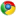Google Chrome 110.0.0.0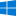Windows 10 x64 Edition

21点的例子没改成every1.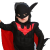任侠说道：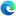Microsoft Edge 110.0.1587.50Windows 10 x64 Edition

已更新。去看 MDN 吧

2.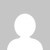Ashishao说道：Google Chrome 55.0.2883.87Windows 10 x64 Edition

第五个 push 应该返回 长度是 4 不是 2

1.任侠说道：Google Chrome 66.0.3359.139Windows 10 x64 Edition

感谢，已更正